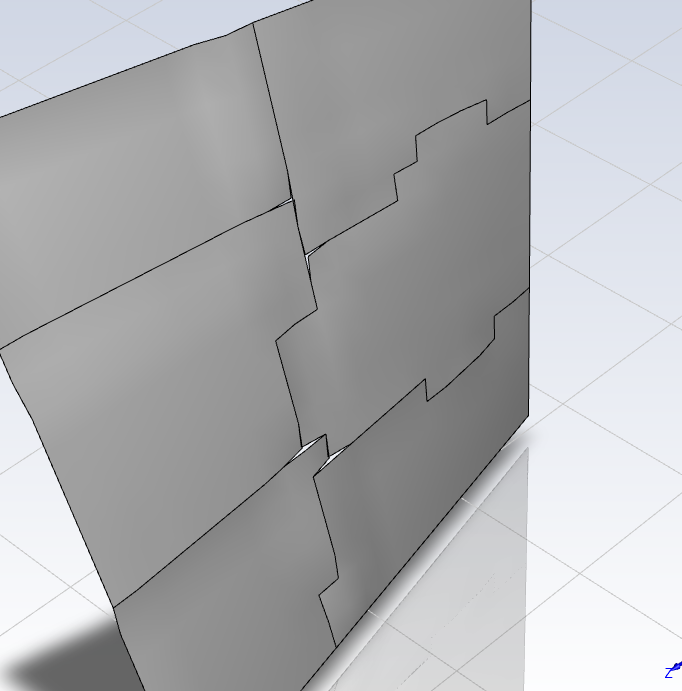# calculating node value from neighbor cell or face by UDF

 Register Blogs Members List Search Today's Posts Mark Forums ReadMay 7, 2022, 08:12calculating node value from neighbor cell or face by UDF #1 New Member   Kevin Liu Join Date: May 2022 Posts: 2 Rep Power: 0Here I want to calculate the node value from neighbor cell or face by UDF. So that I can use this varible to move the grid. [Below is my code.] I'm trying to move the node by location of face center. I save the displacement to N_UDMI. However, in parallel model, for nodes on partition boundary, N_UDMI will have different value because begin_f_loop looped different face, which will lead to separation of nodes as shown in the figure. I need a same N_UDMI for nodes on partition boundaries. For phase changing problem, it should be averaged temperature of neighbor faces. Is there any macro can do this ?#include "udf.h" #include "para.h" #include "sg.h" #include "mem.h" DEFINE_ADJUST(my_adjust_interface_crystal,d) { face_t f; Node *v; Node *v_shadow; int ID = 18; /*ID of interface_crystal*/ Thread *tf = Lookup_Thread(d, ID); int n; real face_x, face_y, face_z; //location of face center real node_x, node_y, node_z; //location of node real x[ND_ND]; begin_f_loop(f,tf) { F_CENTROID(x,f,tf); face_x=x; face_y=x; face_z=x; f_node_loop(f,tf,n) { v=F_NODE(f,tf,n); node_x=NODE_X(v); node_y=NODE_Y(v); node_z=NODE_Z(v); //N_UDMI(v,0) = -0.1*node_y*node_z; //displacement calculated by node location N_UDMI(v,0) = -0.1*face_y*face_z; //displacement calculated by face-center location //Message("\nmyid=%d",myid); } } end_f_loop(f,tf) } DEFINE_GRID_MOTION(grid_motion_crystal,d,dt,time,d time) { Thread *tf = DT_THREAD(dt); face_t f; Node *v; Node *v_shadow; int n; real node_x, node_y, node_z; //location of node SET_DEFORMING_THREAD_FLAG(THREAD_T0(tf)); begin_f_loop(f,tf) { f_node_loop(f,tf,n) { v = F_NODE(f,tf,n); node_x=NODE_X(v); node_y=NODE_Y(v); node_z=NODE_Z(v); if(NODE_POS_NEED_UPDATE(v)) { NODE_POS_UPDATED(v); Message("\nmyid=%d, node_x=%g, node_y=%g, node_z=%g, N_UDMI=%g",myid,node_x,node_y,node_z,N_UDMI(v,0)); //output the node location and the related displacement NODE_X(v) = NODE_X(v) + N_UDMI(v,0); // adjust node by UDM } } } end_f_loop(f,tf) } DEFINE_GRID_MOTION(grid_motion_melt,d,dt,time,dtim e) { Thread *tf = DT_THREAD(dt); face_t f; Node *v; Node *v_shadow; int n; SET_DEFORMING_THREAD_FLAG(THREAD_T0(tf)); begin_f_loop(f,tf) { f_node_loop(f,tf,n) { v = F_NODE(f,tf,n); v_shadow = F_NODE_SHADOW(f,tf,n); if(NODE_POS_NEED_UPDATE(v)) { NODE_POS_UPDATED(v); NODE_X(v) = NODE_X(v) + N_UDMI(v_shadow,0); // adjust node by UDM of shadow node } } } end_f_loop(f,tf) }May 7, 2022, 21:57We can get node value by some macro #2 New Member   Kevin Liu Join Date: May 2022 Posts: 2 Rep Power: 0The answer is found in http://www.eureka.im/280.html Related code is bolded. I have test that it can be use in DEFINE_ADJUST and DEFINE_EXECUTE_AT_END. If you want to have node value of User Defined Face Memory. You need to translate it into User Defined Cell Memory. (can be found in UDF manual), or you will get zero value. Because face memory is not available for postprocessing. It is sometimes necessary to retrieve the values of specific variables at the nodes of your mesh. This could be done by looping over cells/faces and accessing the interpolated data at the nodes. The UDF (DEFINE_ON_DEMAND) included in this solution provides an example for accessing pressure data on the nodes of a boundary surface. Before executing the UDF, please make sure: 1. Data is available (case is initialized/ solved/ data file is read) 2. The correct boundary zone ID is provided 3. The correct field variable name is provided /************************************************** *************************** * UDF to access field variable data at nodes for a face zone ************************************************** **************************/ #include "udf.h" #include "dx.h" #include "mem.h" #define ID 3 /* Zone ID (in boundary condition panel) on which node data is to be obtained */ char *what = "pressure"; /* field variable name for which data is to be extracted */ /* For single phase cases, the names of the field variables can be obtained from FLUENT TUI mode. Example: /display/contours/ => this lists all the field variables that are available. */ /* For multi phase cases, the names of the field variables can be obtained from FLUENT TUI mode. Example: /display/contours/phase-x => this lists all the field variables (y) that are available. The filed variable name = "phase-x-y" => x = phase number ( 1, 2 etc.), y = variable name displayed in TUI. */ DEFINE_ON_DEMAND(my_node_values) { real x, y; int n; face_t f; Node *v; Domain *d = Get_Domain(1); Thread *t = Lookup_Thread(d, ID); FILE *fp = fopen("test_file.txt", "w"); Node_Function_Values(d, what); /* Initalize all node marks on the thread == 0 */ begin_f_loop(f, t) { f_node_loop(f, t, n) { v=F_NODE(f,t,n); NODE_MARK(v) = 0; } } end_f_loop(f, t); /* Loop over the nodes and get the data */ begin_f_loop(f, t) { f_node_loop(f, t, n) { v = F_NODE(f,t,n); x = NODE_X(v); y = NODE_Y(v); if(NODE_MARK(v) == 0) /* if not already visited */ fprintf(fp, "%gt%gt%14.8En", x , y, NODE_VALUE(v)); NODE_MARK(v) = 1; } } end_f_loop(f, t); fclose(fp); }Thread Tools Search this ThreadShow Printable VersionEmail this Page Search this Thread: Advanced Search Display ModesLinear ModeSwitch to Hybrid ModeSwitch to Threaded ModePosting Rules You may not post new threads You may not post replies You may not post attachments You may not edit your posts BB code is On Smilies are On [IMG] code is On HTML code is OffTrackbacks are Off Pingbacks are On Refbacks are On Forum RulesSimilar Threads Thread Thread Starter Forum Replies Last Post vinz OpenFOAM Running, Solving & CFD 286 June 13, 2022 02:48 [blockMesh] Internal walls of zero thickness anger OpenFOAM Meshing & Mesh Conversion 23 February 6, 2020 18:25 [blockMesh] BlockMesh FOAM warning gaottino OpenFOAM Meshing & Mesh Conversion 7 July 19, 2010 14:11 [blockMesh] BlockMeshmergePatchPairs hjasak OpenFOAM Meshing & Mesh Conversion 11 August 15, 2008 07:36 [Commercial meshers] Converting meshes that includes interfaces ham OpenFOAM Meshing & Mesh Conversion 29 January 8, 2007 08:58

All times are GMT -4. The time now is 14:45.